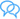﻿ Newton didn't give second law of motion, says new paper | ummid.comWelcome Guest! You are here: Home » Sceince & Technology
Newton didn't give second law of motion, says new paper
Sunday September 21, 2014 10:26 PM, IANS

In Isaac Newton's time, the terms "acceleration" and "second derivative" did not exist, so he could not have deduced F=ma, the second law of motion. This has been unscientifically credited to Newton, says a research paper.

The paper "Isaac Newton, Leonhard Euler and F=ma" was written by Ajay Sharma, assistant director for education with the Himachal Pradesh government.

"Newton gave a velocity dependent force and Euler an acceleration dependent force. If the magnitudes of equations of force F=ma and F=2ma are equal, then it implies 1=2, which is not true," said the paper published this month in international journal "Physics Essays".

"Newton did not discover F=ma in any edition of the 'Principia'. In Newton's time, acceleration and second derivatives were not given. So it was impossible for Newton to write F=ma," Sharma told IANS.

According to him, F=ma was given by Euler in 1775. Due to unknown reasons, it was attributed to Newton.

Sharma, whose research on Newton's law of motion got accolades from Washington-based Euler Society in July, said that after Euler's death in 1783, his law became Newton's second law of motion, which is not scientific.

"There were no second derivative and acceleration at the time of Newton. So he could have not discovered the mathematical form of the second law of motion," Robert E. Bradley, president of the Euler Society, said in a letter to 50-year-old Sharma.

Sharma's 340-page book "Beyond Newton and Archimedes" tries to prove that Newton did not discover the second law of motion.

The book says: Consider a boy is standing at distance of 10 metres from a wall. The boy holds a rubber ball and a cloth ball in his hands.

Firstly, the boy throws the rubber ball with force 2N (Newton) on the wall. The rubber ball after striking the wall rebounds to 10 metres. Thus, action and reaction are equal in this case.

Secondly, the boy throws the cloth ball with an equal 2N force on the wall. The cloth ball rebounds to five metres. Thus action and reaction are not equal.

"Thus to every action, there is an opposite reaction but it may or may not be equal to the action," says the 10th chapter of Sharma's book.

Newton stated in Propositions in Book III of the Principia regarding force of attraction between various heavenly bodies. These are regarded as the law of gravitation.

But Newton did not give any mathematical equation to the law of gravitation, said Sharma, who is working on the basic laws for the past 31 years.Comments| Quick links